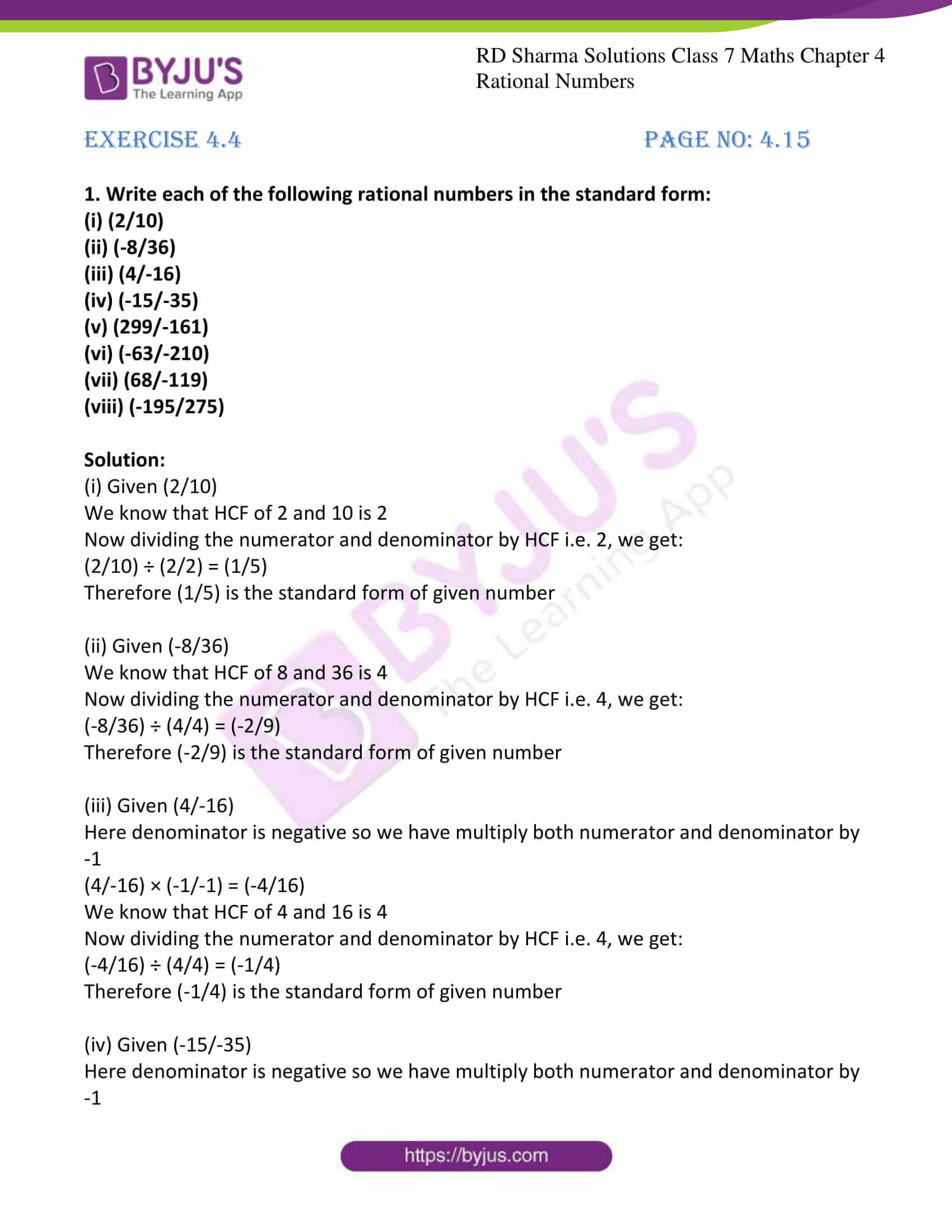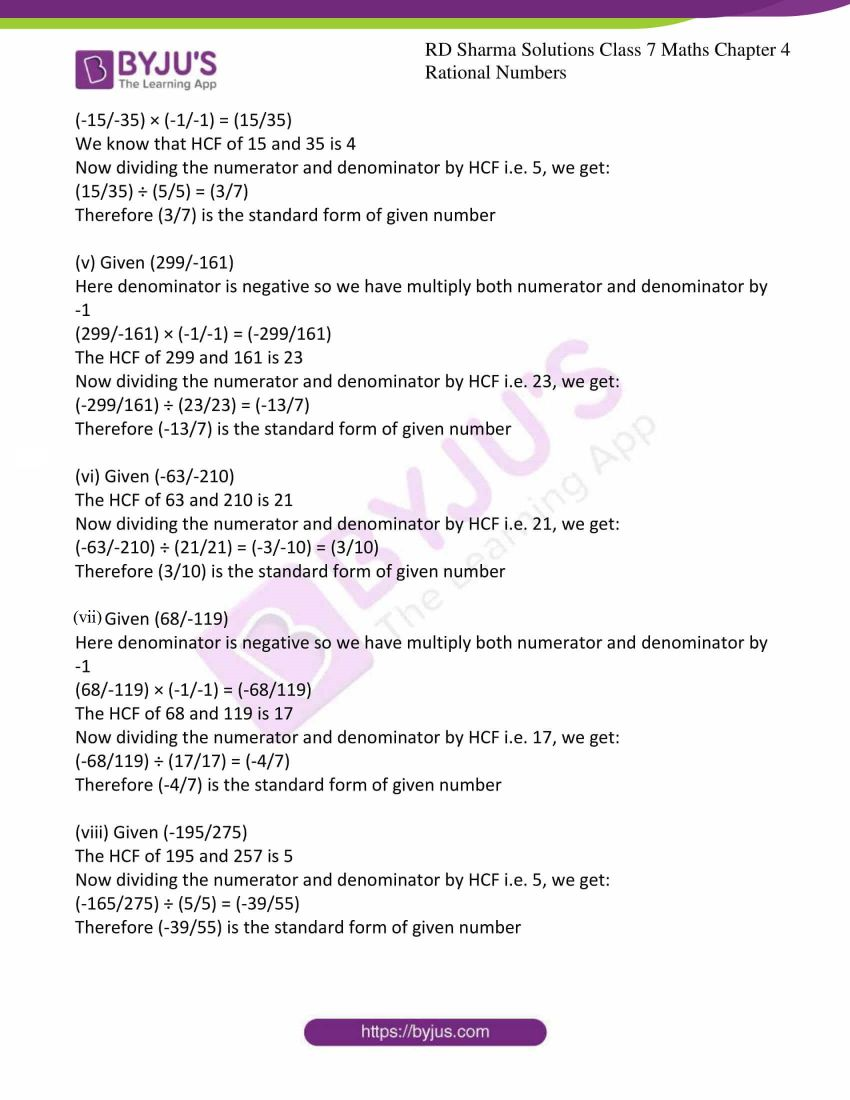# RD Sharma Solutions For Class 7 Maths Exercise 4.4 Chapter 4 Rational Numbers

Students can download the PDF of RD Sharma Solutions for Class 7 Exercise 4.4 chapter 4 from the below links. These solutions have been designed by Mathematics experts at BYJU’S with one hundred per cent accuracy. Knowing the concepts will help students to prepare effectively for their exam and it is prepared by the CBSE board as per the guidelines. This exercise discusses with the standard form of rational numbers. A rational number p/q is said to be in the standard form if p is positive, and the integers p and q have no common divisor other than 1. By practising RD Sharma Solutions for Class 7 students can procure excellence in board exams.

## Download the PDF of RD Sharma Solutions For Class 7 Maths Chapter 4 – Rational Numbers Exercise 4.4### Access answers to Maths RD Sharma Solutions For Class 7 Chapter 4 – Rational Numbers Exercise 4.4

1. Write each of the following rational numbers in the standard form:

(i) (2/10)

(ii) (-8/36)

(iii) (4/-16)

(iv) (-15/-35)

(v) (299/-161)

(vi) (-63/-210)

(vii) (68/-119)

(viii) (-195/275)

Solution:

(i) Given (2/10)

We know that HCF of 2 and 10 is 2

Now dividing the numerator and denominator by HCF i.e. 2, we get:

(2/10) ÷ (2/2) = (1/5)

Therefore (1/5) is the standard form of given number

(ii) Given (-8/36)

We know that HCF of 8 and 36 is 4

Now dividing the numerator and denominator by HCF i.e. 4, we get:

(-8/36) ÷ (4/4) = (-2/9)

Therefore (-2/9) is the standard form of given number

(iii) Given (4/-16)

Here denominator is negative so we have multiply both numerator and denominator by -1

(4/-16) × (-1/-1) = (-4/16)

We know that HCF of 4 and 16 is 4

Now dividing the numerator and denominator by HCF i.e. 4, we get:

(-4/16) ÷ (4/4) = (-1/4)

Therefore (-1/4) is the standard form of given number

(iv) Given (-15/-35)

Here denominator is negative so we have multiply both numerator and denominator by -1

(-15/-35) × (-1/-1) = (15/35)

We know that HCF of 15 and 35 is 4

Now dividing the numerator and denominator by HCF i.e. 5, we get:

(15/35) ÷ (5/5) = (3/7)

Therefore (3/7) is the standard form of given number

(v) Given (299/-161)

Here denominator is negative so we have multiply both numerator and denominator by -1

(299/-161) × (-1/-1) = (-299/161)

The HCF of 299 and 161 is 23

Now dividing the numerator and denominator by HCF i.e. 23, we get:

(-299/161) ÷ (23/23) = (-13/7)

Therefore (-13/7) is the standard form of given number

(vi) Given (-63/-210)

The HCF of 63 and 210 is 21

Now dividing the numerator and denominator by HCF i.e. 21, we get:

(-63/-210) ÷ (21/21) = (-3/-10) = (3/10)

Therefore (3/10) is the standard form of given number

(vii) Given (68/-119)

Here denominator is negative so we have multiply both numerator and denominator by -1

(68/-119) × (-1/-1) = (-68/119)

The HCF of 68 and 119 is 17

Now dividing the numerator and denominator by HCF i.e. 17, we get:

(-68/119) ÷ (17/17) = (-4/7)

Therefore (-4/7) is the standard form of given number

(viii) Given (-195/275)

The HCF of 195 and 257 is 5

Now dividing the numerator and denominator by HCF i.e. 5, we get:

(-165/275) ÷ (5/5) = (-39/55)

Therefore (-39/55) is the standard form of given number